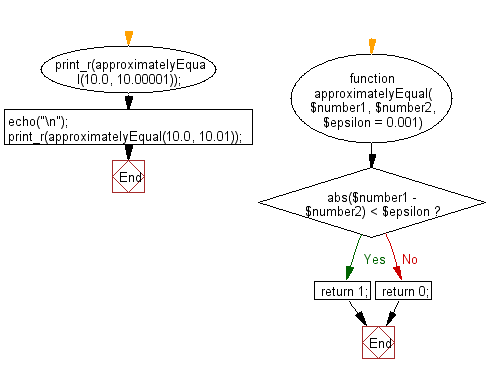﻿ PHP Exercise: Check if two numbers are approximately equal to each other - w3resource

# PHP Exercises: Check if two numbers are approximately equal to each other

## PHP: Exercise-94 with Solution

Write a PHP program to check if two numbers are approximately equal to each other.

Note: Use abs() to compare the absolute difference of the two values to epsilon. Omit the third parameter, epsilon, to use a default value of 0.001.

Sample Solution: -

PHP Code:

``````<?php
//Licence: https://bit.ly/2CFA5XY
function approximatelyEqual(\$number1, \$number2, \$epsilon = 0.001)
{
if (abs(\$number1 - \$number2) < \$epsilon)
return 1;
else
return 0;
}
print_r(approximatelyEqual(10.0, 10.00001));
echo("\n");
print_r(approximatelyEqual(10.0, 10.01));

?>
```
```

Sample Output:

```1
0```

Flowchart:PHP Code Editor:

Have another way to solve this solution? Contribute your code (and comments) through Disqus.

What is the difficulty level of this exercise?

Test your Programming skills with w3resource's quiz.

﻿

## PHP: Tips of the Day

Returns all elements in an array except for the first one

Example:

```<?php
function tips_tail(\$items)
{
return count(\$items) > 1 ? array_slice(\$items, 1) : \$items;
}

print_r(tips_tail([1, 5, 7]));
?>
```

Output:

```Array
(
 => 5
 => 7
)
```